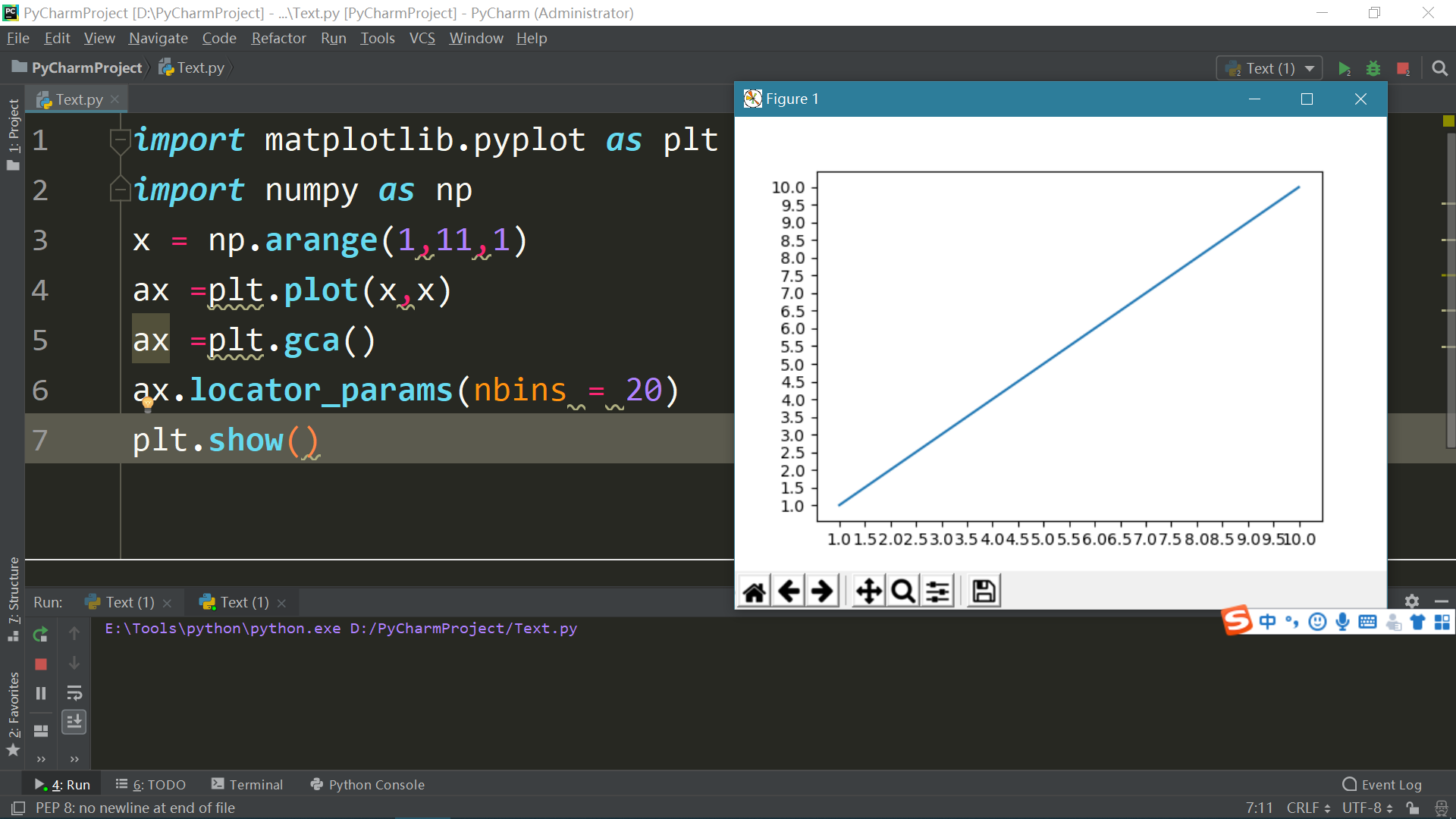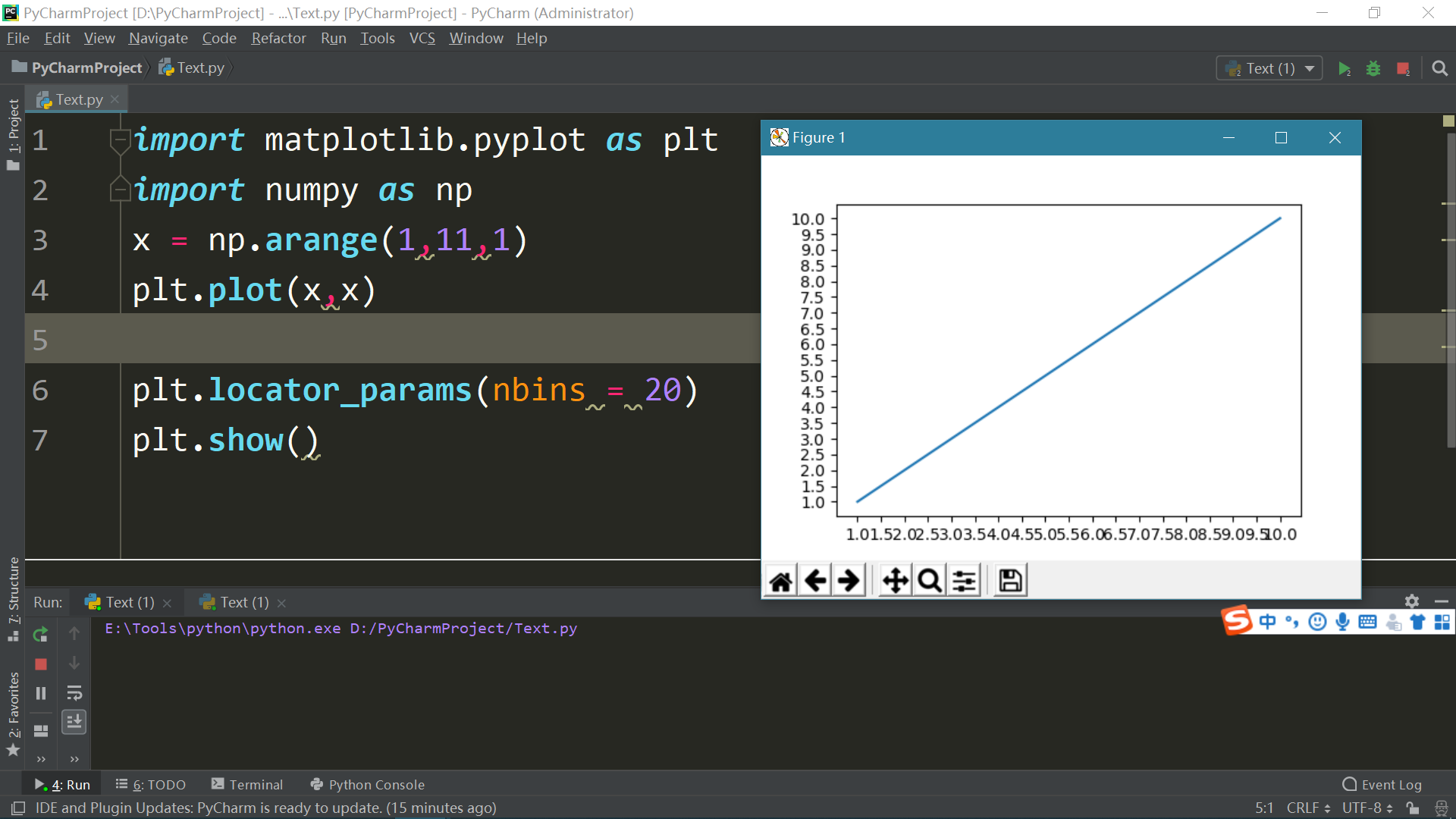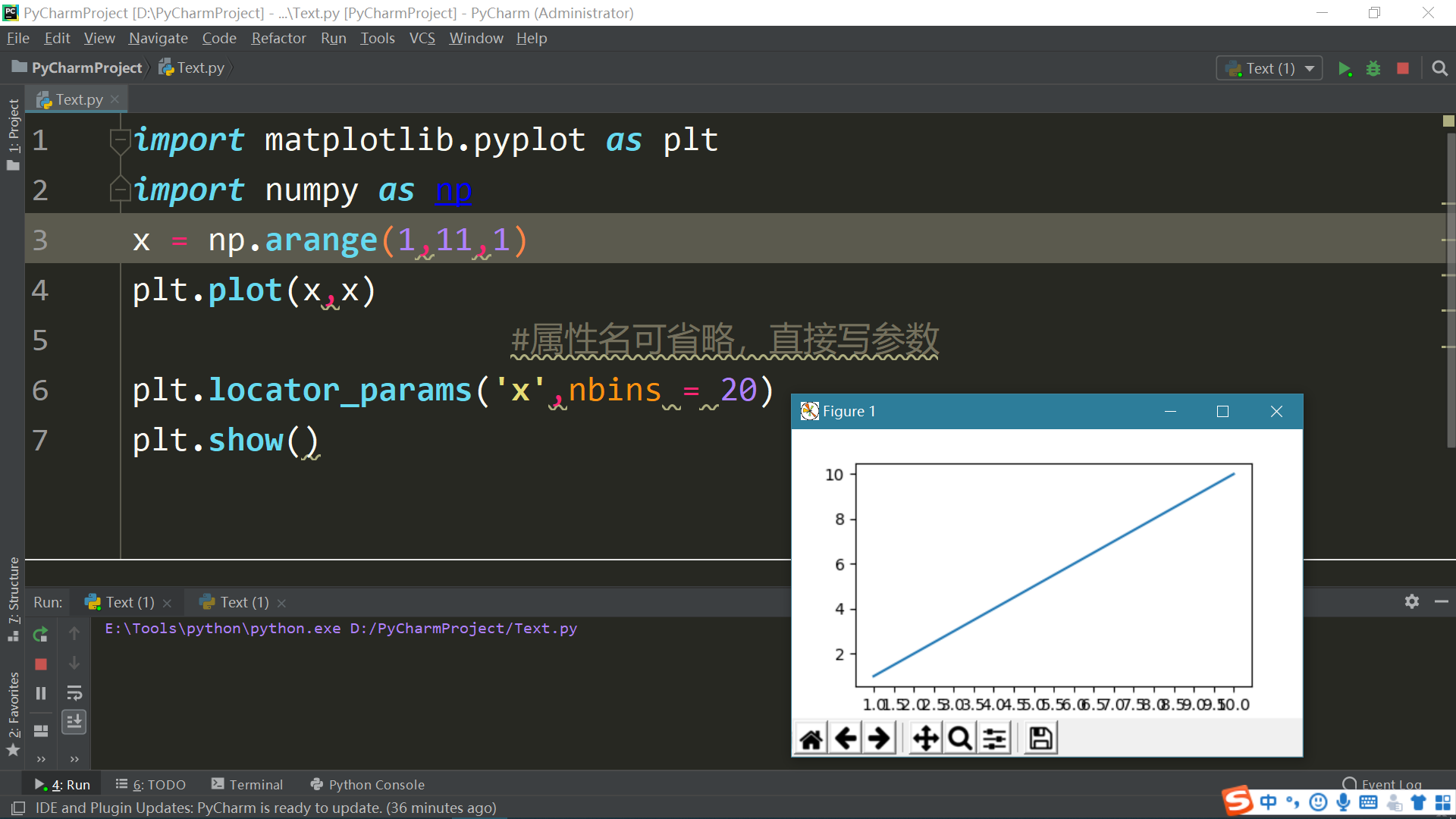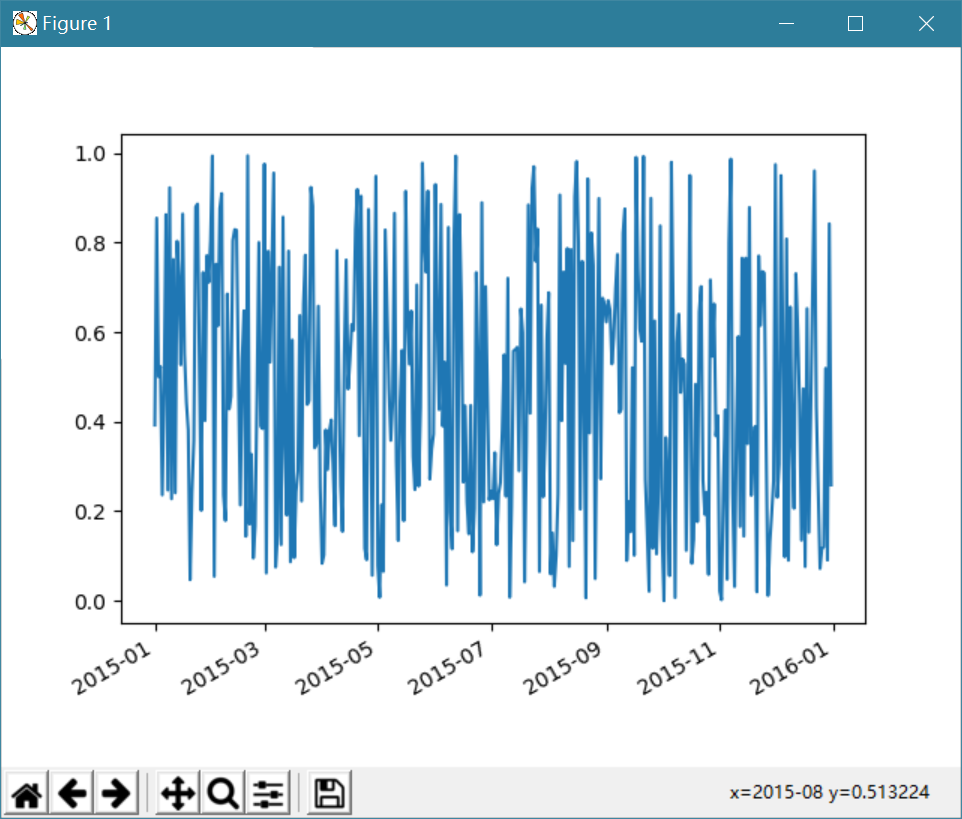Python学习笔记(matplotlib篇)--坐标轴刻度

Python学习笔记--坐标轴刻度

参靠视频:《Python数据可视化分析 matplotlib教程》链接：https://www.bilibili.com/video/av6989413/?p=6

IDE:Pycharm

Python环境：python3.7

Matplotlib:   Matplotlib 1.11

Numpy：  Numpy1.15.

Datetime ：Datetime

• 概念
• 当需要把x，y坐标轴刻度调整的更密集些或者更宽松点
• 学习如何调整x，y坐标轴刻度
• 坐标轴刻度调整
• 　面向对象形式• pyplort形式• locater_params介绍
•  复习面向对象形式，pyplot的区别
• 面向对象形式：面向对象形式不能实时与界面进行交互，在python console修改图表不会立即生效，需要重新运行
• pyplot形式：pyplot形式可以实时与界面交互，在python console修改图表会立即生效，不需要重新运行
• 如果想改变只改变其中一个坐标轴的刻度
• 　更改locater_params中的axis属性（参见上午该属性介绍）• 　当坐标轴显示的是日期时，调整刻度

import matplotlib.pyplot as plt
import numpy as np
import matplotlib as mpl
import datetime
#面向对象方式
fig = plt.figure()
start = datetime.datetime(2015,1,1)#起始时间
stop = datetime.datetime(2016,1,1)#停止时间
delta = datetime.timedelta(days=1)
dates = mpl.dates.drange(start,stop,delta)#生成一个matplotli认得的days序列
y = np.random.rand(len(dates))
ax = plt.gca()
ax.plot_date(dates,y,linestyle = '-',marker = '')
date_format = mpl.dates.DateFormatter('%Y-%m')
#只显示年月
ax.xaxis.set_major_formatter(date_format,)
fig.autofmt_xdate()
#开启自适应
plt.show()
• 　效果• 结语：

感谢matplotlib，numply提供的文档，感谢麦子学院提供的视频教学

posted @ 2018-09-17 17:08  9974  阅读(30544)  评论(0编辑  收藏  举报